Addition of integers using number line

Chapter 6 Class 6 Integers
Concept wise

#### Add 3 & 5 on number line

3 + 5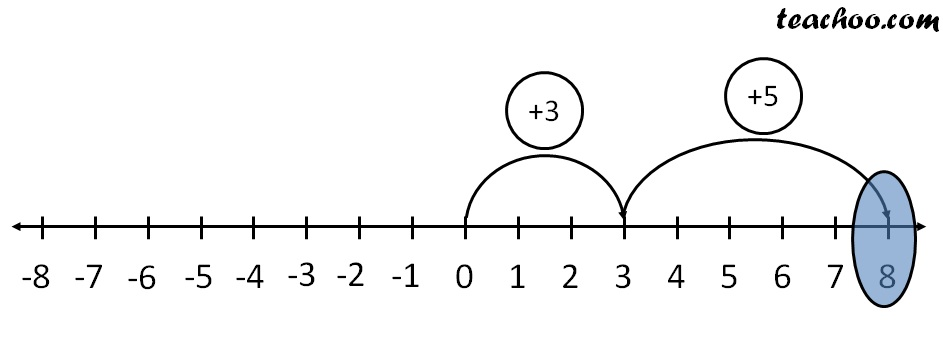First we move 3 steps to the right from 0

And then , we move 5 steps to the right

we reach

∴ 3 + 5 = 8

#### Add (−3) & (−5) on number line

(−3) + (−5)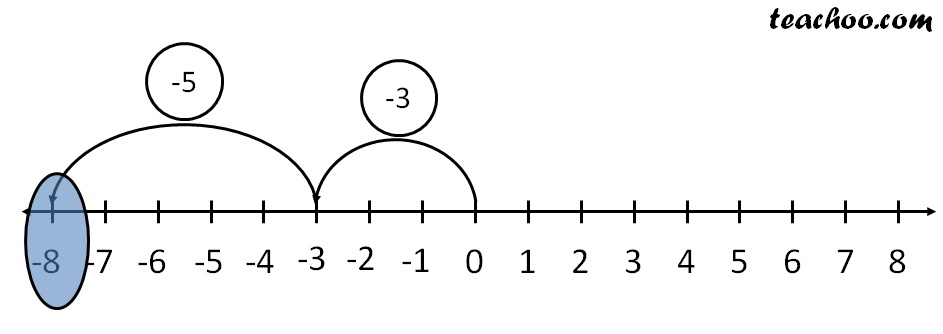First we move 3 steps to the left from 0

And then , we move 5 steps to the left

we reach −8

∴   (−3) + (−5) = −8

#### Add (−3) & (5) on number line

(−3) + (5)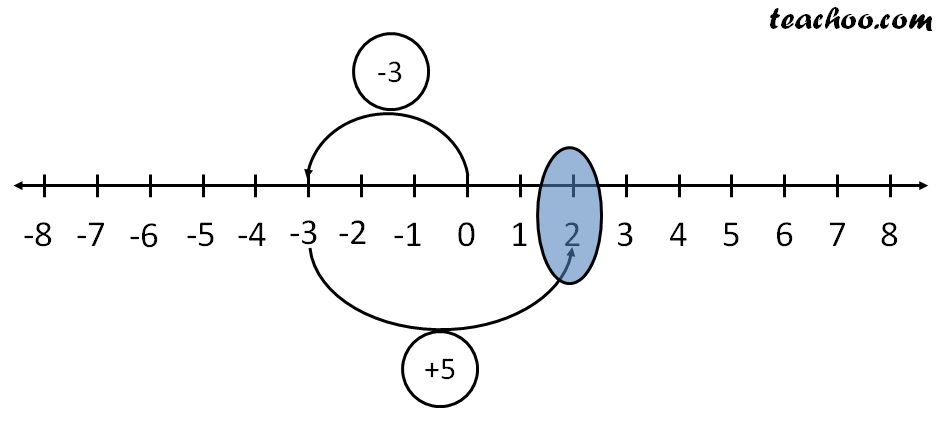First we move 3 steps to the left from 0

And then , we move 5 steps to the right

we reach 2

∴   (−3) +  5  = 2

#### Add (3) & (−5) on number line

3 + (–5)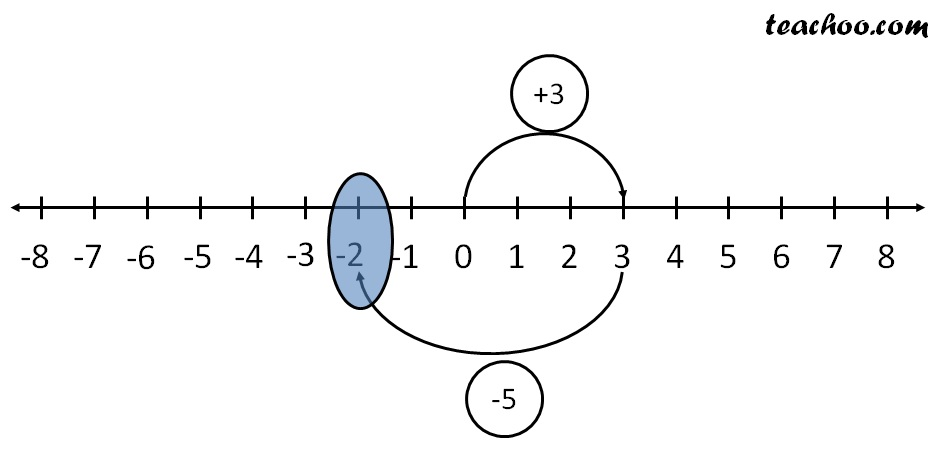First we move 3 steps to the right from 0

And then , we move 5 steps to the left

we reach −2

∴   3 + (-5) = −2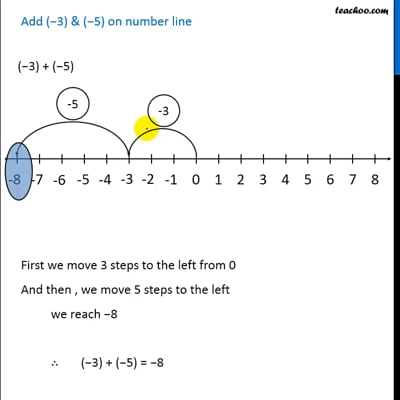This video is only available for Teachoo black users

Get live Maths 1-on-1 Classs - Class 6 to 12